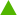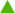44,133 Pages

## What is the Grand Exchange Market Watch?

The Grand Exchange Market Watch is a project set up to monitor and record the prices and other information on items on the Grand Exchange.

## Who created this project?

This project was thought up and originally coded by Robert Horning. Many other people over the years since it was created have been involved in "tweaking" the organization of this project and have contributed a huge amount of information to make everything work and to keep the information up to date.

## How do I update prices?

Usually, users do not need to manually update the Grand Exchange Market Watch as bots keep it up to date. However, the bots require several hours before all prices can be updated.

If you require updated prices urgently, we provide an option of manually updating individual price data. On top of each Exchange page, there is a button called "Update price". Clicking the button will update the price automatically. Note that if you are new to our wiki and cannot see the Update button, continue editing for a few days until you become an autoconfirmed user.

A complete list of items needing price updates can be found in Category:Needs price update. If you are still confused, use our test price updater.

### List of bots

Below is the list of bots currently involved in the project (as of February 2013):

## What is an index?

An index is a method of measuring the value of a section of the market. It is computed from the prices of selected items. In a price-weighted index such as the Dow Jones Industrial Average (DJIA), the price of each component item is the only consideration when determining the value of the index. Items may be removed or added to indices over time, but the index will remain "neutral" when that happens, following the method that the Wall Street Journal uses when the DJIA is adjusted (see below).

The Common Trade Index (CTI) is one of the indices calculated from the Grand Exchange Market Watch. The CTI shows the health of the RuneScape economy in general. The CTI is worked out using a list of items which are traded very often. There are also other indices such as the Discontinued Rare, Rune, Log, Food, Metal, Herb and Indices, all of which show the health of that aspect of the RuneScape economy and work in the same way as the Common Trade Index.

The Common Trade Index is displayed as 2 numbers. The first is the index number. This shows the comparative prices of all the items on the list compared to their price on the base date. A value above 100 means that in general the prices have risen and consequently that the RuneScape economy is doing quite well, while a value below 100 means that overall prices have dropped.

Next to that number is index change number accompanied by an arrow, either red or green. This shows whether the index has gone up or down since it was last calculated, a negative number and a red arrow indicate that the index has dropped as prices have generally fallen, while a positive number shows that the prices have generally risen.

### List of indices

Index Current Change Base date Last adjustment date Number of items Index divisor
Common Trade Index 158.11+0.43 9 December 2007 12 February 2012 27 23.9535
Discontinued Rare Index 4,921.30+0.00 31 December 2008 30 December 2014 24 15.6373
Rune Index 573.23+0.30 15 December 2007 30 August 2014 21 21.2740
Log Index 829.87+4.95 19 December 2007 8 November 2013 11 10.3117
Food Index 455.18-1.34 12 January 2008 20 May 2015 15 8.7619
Metal Index 309.89+3.34 10 December 2007 17 17.0000
Herb Index 393.10-1.81 9 June 2009 6 November 2011 34 33.2409

## How is an index calculated?

The index is calculated by summing the sum of price ratios (current price of items divided by their prices on the base date), multiplying by 100, and dividing with the "index divisor".

\begin{align} \text{index} & = \sum_{i=1}^{n} \left ( \frac{p_i}{q_i} \right ) \times \frac{100}{{div}_{\text{index}}} \\ & = \left ( \frac{p_1}{q_1} + \frac{p_2}{q_2} + \dots + \frac{p_n}{q_n} \right ) \times \frac{100}{{div}_{\text{index}}} \end{align}

\begin{align} \text{where:} \qquad \qquad p & = \text{current price} \\ q & = \text{base price} \\ n & = \text{number of items} \\ \sum \left ( \frac{p}{q} \right ) & = \text{sum of price ratios} \\ {div}_{\text{index}} & = \text{index divisor} \end{align}

For example, let us assume the index is composed of four fictional items (A=30, B=40, C=70, D=60). At the point of the creation of the index, the index would equal to 100, and the index divisor, would be equal to the number of items included in the index.

\begin{align} \text{index} & = \sum_{i=1}^{n} \left ( \frac{p_i}{q_i} \right ) \times \frac{100}{{div}_{\text{index}}} \qquad \text{for }{A, B, C}\text{ and }{D} \\ & = \left ( \frac{p_1}{q_1} + \frac{p_2}{q_2} + \dots + \frac{p_n}{q_n} \right ) \times \frac{100}{n} \\ & = \left ( \frac{30}{30} + \frac{40}{40} + \frac{70}{70} + \frac{60}{60} \right ) \times \frac{100}{4} \\ & = 100 \end{align}

After several months, the prices of the four items would have changed (A=22, B=31, C=85, D=64). When the index is re-calculated, the index is down to 94.73 by 5.27 points. As long as the items themselves are not changed (via addition and/or removal), the index divisor remains equal to the number of items.

\begin{align} \qquad \qquad \qquad \qquad \text{index} & = \sum_{i=1}^{n} \left ( \frac{p_i}{q_i} \right ) \times \frac{100}{{div}_{\text{index}}} \qquad \text{for }{A, B, C}\text{ and }{D} \\ & = \left ( \frac{22}{30} + \frac{31}{40} + \frac{85}{70} + \frac{64}{60} \right ) \times \frac{100}{4} \\ & = 94.73 \end{align}

## What happens when index items need to be changed?

When old items are removed and/or new items are added to the index, the index is adjusted accordingly. The index divisor needs to be re-calculated and is no longer equal to the total number of items. While the value of the index remains the same at the moment of the removal and/or addition, the index divisor is different.

\begin{align} \text{since:} \qquad \qquad \qquad \qquad \text{index}_{\text{adj}} & = \text{index}_{\text{old}} \\ \sum \left ( \frac{p}{q} \right )_{\text{adj}} \times \frac{\cancel{100}}{{div}_{\text{adj}}} & = \sum \left ( \frac{p}{q} \right )_{\text{old}} \times \frac{\cancel{100}}{{div}_{\text{old}}} \\ \frac{\sum \left ( \frac{p}{q} \right )_{\text{adj}}}{{div}_{\text{adj}}} & = \frac{\sum \left ( \frac{p}{q} \right )_{\text{old}}}{{div}_{\text{old}}} \\ \end{align}

$\text{thus:}$
${div}_{\text{adj}} = {div}_{\text{old}} \times \frac{\sum \left ( \frac{p}{q} \right )_{\text{adj}}}{\sum \left ( \frac{p}{q} \right )_{\text{old}}}$

$\text{where:}$

\begin{align} {div}_{\text{old}} & = \text{index divisor prior to adjustment} \\ {div}_{\text{adj}} & = \text{index divisor after adjustment} \\ \sum \left ( \frac{p}{q} \right )_{\text{old}} & = \text{sum of price ratios prior to adjustment} \\ \sum \left ( \frac{p}{q} \right )_{\text{adj}} & = \text{sum of price ratios after adjustment} \\ & = \text{sum of price ratios prior to adjustment} \\ & \quad - \text{sum of removed ratios} + \text{sum of added ratios} \\ & = \sum \left ( \frac{p}{q} \right )_{\text{old}} - \sum \left ( \frac{p}{q} \right )_{\text{removed}} + \text{number of added items} \\ \end{align}

From the earlier example of the fictional index with four items (see above), Item B will be removed, and new items E and F are added (E=120, F=354).

${div}_{\text{old}} = 4$
$\sum \left ( \frac{p}{q} \right )_{\text{old}} = \left ( \frac{22}{30} + \frac{31}{40} + \frac{85}{70} + \frac{64}{60} \right ) = 3.78928571 \qquad \left ( \text{for }{A, B, C}\text{ and }{D} \right )$
$\sum \left ( \frac{p}{q} \right )_{\text{adj}} = 3.78928571 - \left ( \frac{31}{40} \right ) + 2 = 5.01428571 \qquad \left ( \text{for }{A, C, D, E}\text{ and }{F} \right )$
${div}_{\text{adj}} = 4 \times \frac{5.01428571}{3.78928571} = 5.2931$

To check if our calculations are correct, we need to check the "adjusted" index using the "adjusted" index divisor.

$\text{index}_{\text{old}} = 94.73$
$\text{index}_{\text{adj}} = \left ( \frac{22}{30} + \frac{85}{70} + \frac{64}{60} + \frac{120}{120} + \frac{354}{354} \right ) \times \frac{100}{5.2931} = 94.73$